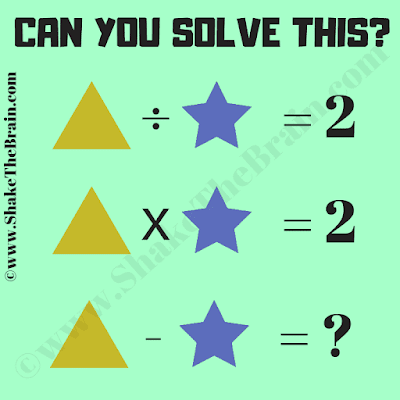## Sunday, May 20, 2018

This Mathematical Equations Brain Teaser is for students. In this Picture Puzzle, there are many different Mathematical equations in which variable are represented by different geometrical shapes. Can you find the values of these shape variables and then solve the last equation?Can you solve this maths brain teaser?

Answer of this "Mathematical Equations Brain Teaser for Students", can be viewed by clicking on the button. Please do give your best try before looking at the answer.

1.1.